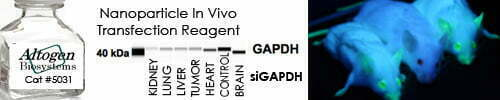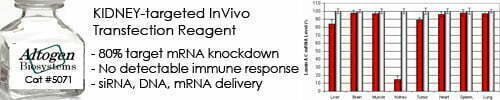••••Shop Products

# ssDNA: Mass to Moles Convertor

The ssDNA (single-stranded DNA) mass to moles converter is a tool that calculates the number of moles of ssDNA based on its mass in grams and its length in nucleotides. The conversion involves taking into account the molecular weight of the ssDNA, which depends on the number and type of nucleotides in the sequence.

The formula for converting ssDNA mass to moles is:

moles = mass / (length x 330)

Where:

• mass is the mass of ssDNA in grams
• length is the length of the ssDNA in nucleotides
• 330 is the average molecular weight of a nucleotide in the ssDNA sequence, in grams per mole.

To use the ssDNA mass to moles converter, you need to input the values of the mass of ssDNA and its length in nucleotides, and the calculator will calculate the corresponding number of moles for you. The resulting mole value can be useful in various applications in molecular biology and biotechnology, such as determining the concentration of ssDNA in a sample, designing primers for PCR amplification, or calculating the amount of ssDNA needed for a particular experiment.

ssDNA: Mass to Moles Calculator
Enter DNA length:
nt
Enter DNA mass:
μg

Sorry, comments are closed for this post.